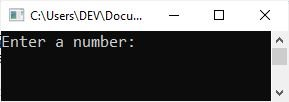# C++ Formatting Output

This article will teach you how to format the output console. In other words, this article discusses information that can be used to design the output console so that the user can easily read the data. And, on occasion, we must format the output in order to lay out the data in a way that meets the requirements. So without any further delay, let's start.

Formatting output in C++ is important in the development of the output screen, which can be easily read and understood. C++ offers the programmer several input/output manipulators. Two of these widely used I/O manipulators are:

In order to use these manipulators, you must include the header file named iomanip. Here is an example showing how to include this header file in your C++ program.

`#include<iomanip>`

## The setw() manipulator in C++

In C++, the setw() manipulator sets the width of the field assigned for the output. It takes the size of the field (in number of characters) as a parameter. Here is an example of this code fragment:

`cout<<setw(6)<<"C";`

generates the following output on the screen (each underscore represents a blank space).

`_ _ _ _ _C`

The setw() manipulator does not stick from one cout statement to the next. For example, if you want to right-justify three numbers within an 8-space field, you will need to repeat setw() for each value, as shown below:

```cout<<setw(8)<<22<<"\n";
cout<<setw(8)<<4444<<"\n";
cout<<setw(8)<<666666<<endl;```

The output will be (each underscore represents a blank space):

```_ _ _ _ _ _ 2 2
_ _ _ _ 4 4 4 4
_ _ 6 6 6 6 6 6```

Note: The endl and "\n" are both used to break the line.

### Example of formatting output using setw() in C++

Here is an example program demonstrating how to format the output screen using "setw()" in C++.

```#include<iostream>
#include<iomanip>
using namespace std;
int main()
{
int i, num;
cout<<"Enter a number: ";
cin>>num;
cout<<"\nThe multiplication table of "<<num<<" is:\n";
for(i=1; i<=10; i++)
cout<<num<<setw(3)<<"*"<<setw(4)<<i<<setw(4)<<"="<<setw(4)<<num*i<<"\n";
return 0;
}```

The following snapshot shows the initial output produced by the above C++ example program demonstrating the "setw()" method.Now supply the input as a number, say 8, to produce the following output:See how well-organized the output becomes after formatting it. However, if we remove all the "setw()" methods from the above example, that is, replace the last "cout" statement with the following:

`cout<<num<<"*"<<i<<"="<<num*i<<"\n";`

then the output should look like this:

```Enter a number: 8

The multiplication table of 8 is:
8*1=8
8*2=16
8*3=24
8*4=32
8*5=40
8*6=48
8*7=56
8*8=64
8*9=72
8*10=80```

Here is another type of C++ program, also demonstrating output formatting in C++.

```#include<iostream>
#include<iomanip>
using namespace std;
int main()
{
int i;
long int num;
cout<<"Enter a number: ";
cin>>num;
cout<<"\nMultiplying (by 5) and printing the result ten times in three columns:\n";
for(i=0; i<10; i++)
{
cout<<num<<setw(25)<<num<<setw(25)<<num<<"\n";
num = num * 5;
}
return 0;
}```

The following snapshot shows the sample run of this C++ program with the same user input as the previous program's sample run.## The setprecision() manipulator in C++

In C++, the setprecision() manipulator sets the total number of digits to be displayed when floating-point numbers are printed. Here is an example of this code fragment:

`cout<<setprecision(5)<<123.456;`

will print the following output to the screen (notice the rounding):

`123.46`

The setprecision() manipulator can also be used to set the number of decimal places to be displayed. In order for setprecision() to accomplish this task, you will have to set an ios flag. The flag is set with the following statement:

`cout.setf(ios::fixed);`

Once the flag has been set, the number you pass to setprecision() is the number of decimal places you want displayed. The following code

```cout.setf(ios::fixed);
cout<<setprecision(5)<<12.345678;```

generates the following output on the screen (notice no rounding):

`12.34567`

In the statement,

`cout.setf(ios::fixed);`

"fixed," i.e., ios::fixed, is referred to as a format option. Other format alternatives include the following:

Format Value Meaning
left left-justify the output
right right-justify the output
showpoint displays decimal point and trailing zeros for all floating point numbers, even if the decimal places are not needed
uppercase display the "e" in E-notation as "E" rather than "e"
showpos display a leading plus sign before positive values
scientific display floating point numbers in scientific ("E") notation
fixed display floating point numbers in normal notation - no trailing zeroes and no scientific notation

These options can be removed by replacing setf (used with cout; recall cout.setf) with unsetf. For example, to make 5.8 appear as 5.80, the following lines of code are required:

```cout.setf(ios::fixed);
cout.setf(ios::showpoint);
cout<<setprecision(2);
cout<<5.8;```

Please note that all the subsequent couts retain the precision set with the last setprecision(). That means setprecision() is "sticky." Whatever precision you set sticks with the cout device until such time as you change it with an additional setprecision() later in the program.

C++ Quiz

« Previous Tutorial Next Tutorial »